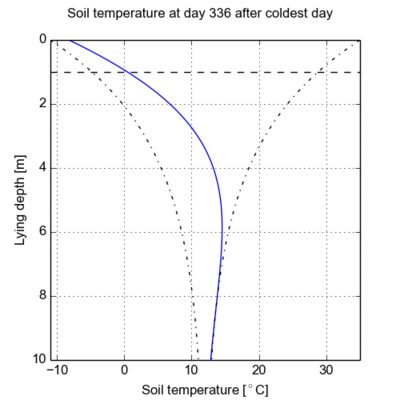# Soil Temperature Calculator

The online soil temperature calculator is based on a simplified mathematical model describing the expected temperature curve in the soil. It is especially useful to estimate the maximum soil temperature at a certain lying depth of buried cables.

Posted 2015-04-20
Categories: Theory, User guides

### Introduction

The soil temperature calculator helps you to estimate the ambient soil temperature at a particular lying depth and day of year. It is based on a mathematical model describing the expected temperature curve in the soil. It is especially useful to estimate the maximum soil temperature at a certain lying depth. The air temperature is assumed to fluctuate sinusoidally over the year, and the soil temperature is damped depending on the thermal diffusivity of the soil.

#### Free Tool

Also have a look at our free Solar Radiation Calculator

### Theory

Cableizer is based on the IEC 60287 standards, which assume a uniform ambient temperature in the soil as initial condition (before the addition of loaded cables and heat sources). In reality, however, the soil temperature may vary depending on the depth and the time of year. In the simplified mathematical model used in the Cableizer soil temperature calculator, the air temperature is assumed to fluctuate sinusoidally over the year, and the soil temperature is damped depending on the thermal diffusivity of the soil according to: $$T(t,z)=T_0-\Delta Te^{-z/z_0}cos(\omega t-z/z_0)$$ where:

 $T_0$ Average annual temperature °C $\Delta T$ Maximum annual temperature variation from average °C $D_h$ Thermal diffusivity of soil m²/day $t$ Number of days after coldest day $z$ Depth of burial m $\omega$ Angular frequency 1/day $2\pi /365$ $z_0$ Damping depth m $\sqrt{2D_h/\omega}$

With the above model, the air temperature is coldest at day 0 with a temperature of $T_0-\Delta T$. Approximately at days 91 and 274, the air temperature corresponds to the average annual temperature $T_0$. Between day 182 and 183, the air temperature is at its peak at a temperature of $T_0+\Delta T$. Depending on the laying depth, however, the peak soil temperature occurs at a delay compared to the peak air temperature, refer to Figure 1. With the Cableizer soil temperature calculator, you can easily estimate the maximum expected soil temperature at a certain laying depth.Fig. 1 Annual soil temperature fluctuations based on the above model.

### Model verification

While a model verification with actual soil temperature is out of the scope of this blog post, the results from the Cableizer soil temperature calculator are compared with results from the geotechnical agency Dr. Mattmüller (in German only). The following results are based on an annual temperature of 9 ± 14 °C, a thermal diffusivity of 4.17·10-7 m²/s, and at day 165 (July 1). The Cableizer soil temperature calculator shows good agreement.

Laying depthDr. MattmüllerCableizer
0 m22 °C22.4 °C
1.5 m12 °C12.4 °C
4 m7.5 °C7.7 °C

As a final remark: The Cableizer soil temperature calculator is equally valid when using temperatures in the unit K.

12
10
9
10
2
9
11
2